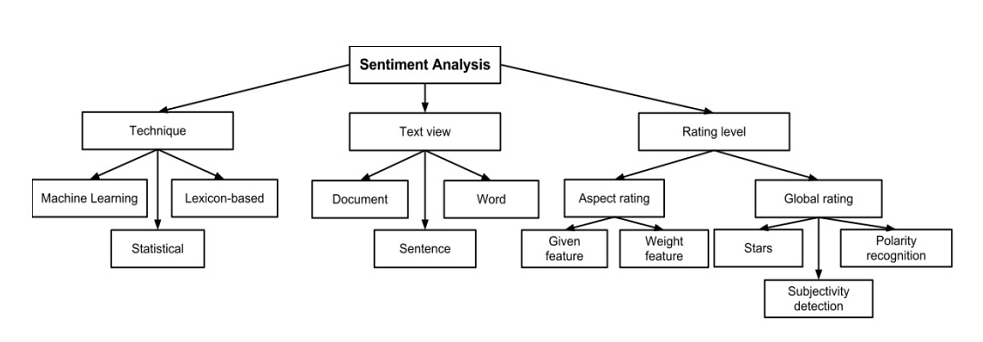## 16.6 Sentiment Analysis

Classification of Sentiment Analysis Methods

16.6 shows various classification of sentiment analysis methods ()Figure 16.6: Classification of Sentiment Analysis Methods

### 16.6.1 Method

• Lexicon (or Dictionary) based method used in the following illustration.

• Sentence level classification

• Eight emotions to classify: “anger, fear, anticipation, trust, surprise, sadness, joy, and disgust”

• Lexicon Used: NRC Emotion Lexicon.

• See for more details on the lexicon.

# required libraries sentiment analysis
library(syuzhet)
library(lubridate)
library(ggplot2)
library(scales)
library(reshape2)
library(dplyr)
library(qdap)
# convert data to dataframe for analysis
data_sent = data_sent[!apply(data_sent, 1, function(x) any(x == "")), ]  #remove rows with empty values
data_sent = data_sent[wc(data_sent$text) > 4, ] #more than 4 words per tweet tw2 = data_sent$text
• Conduct Sentiment Analysis and Visualise
mySentiment = get_nrc_sentiment(tw2)  #this can take some time
tweets_sentiment = cbind(data_sent, mySentiment)
# save the results
saveRDS(tweets_sentiment, file = "tw_sentiment.rds")
• Plot
sentimentTotals = data.frame(colSums(tweets_sentiment[, c(4:13)]))
names(sentimentTotals) = "count"
sentimentTotals = cbind(sentiment = rownames(sentimentTotals), sentimentTotals)
rownames(sentimentTotals) = NULL
# plot
ggplot(data = sentimentTotals, aes(x = sentiment, y = count)) + geom_bar(aes(fill = sentiment),
stat = "identity") + theme(legend.position = "none") + xlab("Sentiment") + ylab("Total Count") +
ggtitle("Sentiment Score for Sample Tweets") + theme_minimal() + theme(axis.text = element_text(size = 15,
face = "bold"))Figure 16.7: Emotions/Sentiment Scores

### References

Collomb, Anaıs, Crina Costea, Damien Joyeux, Omar Hasan, and Lionel Brunie. 2014. “A Study and Comparison of Sentiment Analysis Methods for Reputation Evaluation.”
Mohammad, Saif M, and Peter D Turney. 2010. “Emotions Evoked by Common Words and Phrases: Using Mechanical Turk to Create an Emotion Lexicon.” In Proceedings of the NAACL HLT 2010 Workshop on Computational Approaches to Analysis and Generation of Emotion in Text, 26–34. Association for Computational Linguistics.